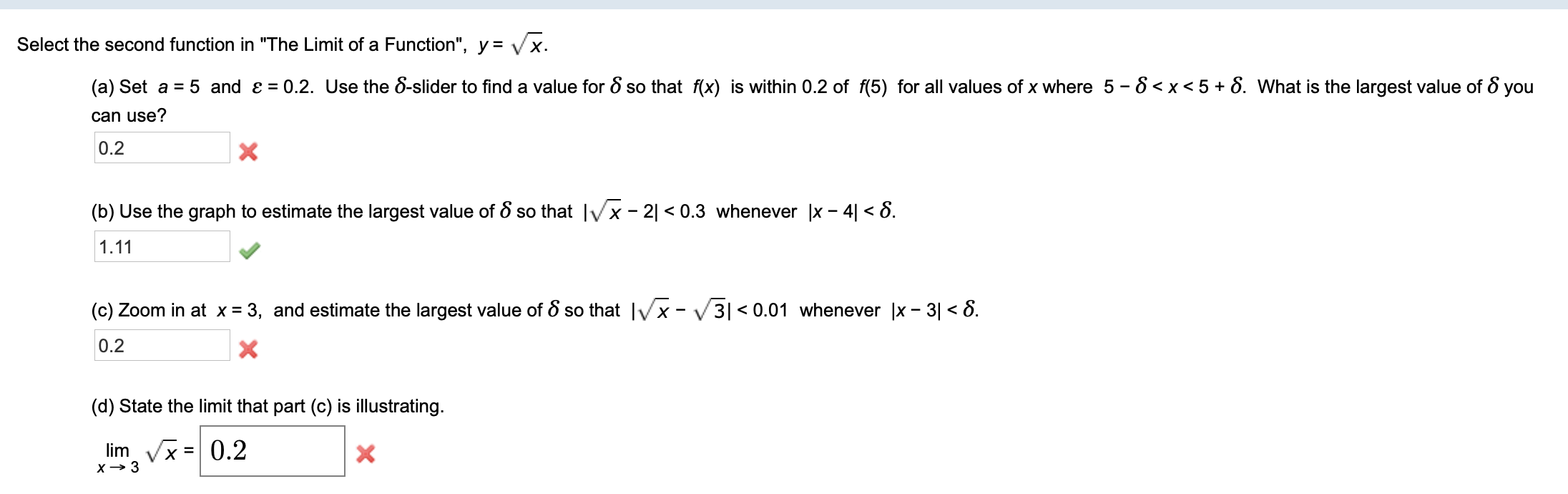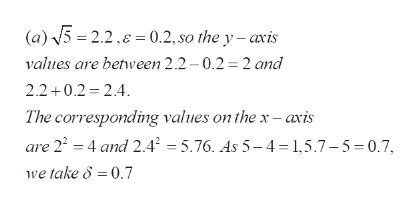Select the second function in "The Limit of a Function", y = Vx0.2. Use the 8-slider to find a value for 8 so that f(x) is within 0.2 of f(5) for all values of x where 5 - 8

Question

please answer parts a, b, and chelp_outlineImage TranscriptioncloseSelect the second function in "The Limit of a Function", y = Vx 0.2. Use the 8-slider to find a value for 8 so that f(x) is within 0.2 of f(5) for all values of x where 5 - 8
Step 1

To estimate the value of delta (as in epsilon - delta definition of limits) using graphing calculator

Step 2

In the solution, we assume that the grid is marked upto the tenth of a unit interval on both axes. For instance, the interval [0,1] is divided into 10 equal parts at 0,0.1,0.2 ,,,,0.9 ,1 .

Step 3

(a) the value of delta is 0.7 , under the assumption th...help_outlineImage Transcriptionclose(a)5 2.2, 0.2, so the y - axis values are between 2.2 0.2 2 and 2.2+0.2 2.4 The corresponding values on the x -axis are 22 4 and 2.42 =5.76. As 5-4 1,5.7-5=0.7, we take 8 =0.7 fullscreen

Want to see the full answer?

See Solution

Want to see this answer and more?

Our solutions are written by experts, many with advanced degrees, and available 24/7

See Solution
Tagged in

Calculus